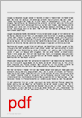# How does the concentration affect the chemical balance?

If you throw a piece of zinc into a test tube filled with dilute hydrochloric acid, hydrogen is produced, which escapes from the test tube and can be collected in a measuring cylinder. The resulting product is lost to the system, so it is a open system. This reaction would also be reversible if the products hydrogen and zinc chloride were to be locked in a closed container at high temperatures and under high pressure. You then get a closed system. In closed systems, a chemical equilibrium is established after some time.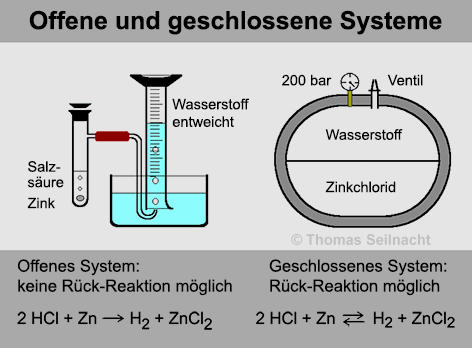This phenomenon should first be explained using the "Apple War" model example: A grandfather is playing a strange game against his grandson in the garden. Each participant receives 9 apples at the beginning. The aim of the game is to throw as many apples as possible into the opposing field. After a while you can see that a constant, dynamic equilibrium is established. There are more apples in the grandfather's field than in the grandson's field.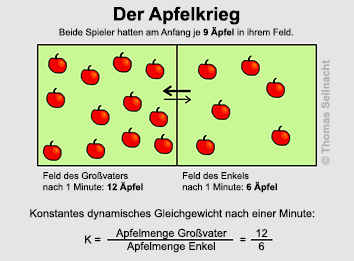Now the equilibrium reaction between iodine and hydrogen can also be better understood. A mixture of gaseous iodine and gaseous hydrogen partially reacts in a closed system at elevated temperature to form hydrogen iodide.

Forward reaction: H2 (g) + I.2 (G)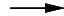2 HI (g) ΔHR. = −10 kJ / mol

The reaction does not take place completely, however, since hydrogen iodide partially decomposes again to iodine and hydrogen at the same temperature:

Back reaction: 2 HI (g)H2 (g) + I.2 (G) ΔHR. = +10 kJ / mol

A chemical equilibrium is established in which all substances involved in the chemical reaction are present in a certain proportion. It doesn't matter whether you start with the raw materials or with the products, the same state of equilibrium is always established.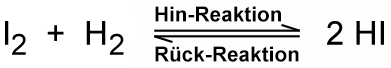The double arrow indicates the equilibrium reaction. In the outward reaction, the concentration of the starting materials steadily decreases, thereby reducing the speed of the outward reaction. At the same time, the concentration of the products increases, thus increasing the speed of the reverse reaction. If the reaction speed of the back and forth reaction is the same, the chemical equilibrium is established.

Law of mass action

An equilibrium constant can be established with a characteristic value at a given temperature. For a chemical reaction by type:

aA + bB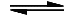cC + dD

the temperature-dependent equilibrium constant or mass action constant K applies: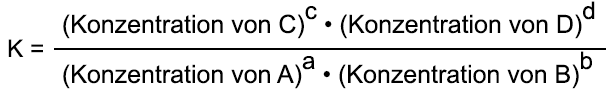When the quotient of the product of the concentrations of the end substances and the product of the concentrations of the starting substances reaches a constant value, the equilibrium state is reached. Guldberg and Waage referred to this law in 1867 as Law of mass action

Calculation exampleWhen iodine and hydrogen react to form hydrogen iodide, a concentration of 3.531 mol / l hydrogen iodide is obtained at a certain temperature. At the same time, the starting materials are present in a concentration of 0.4789 mol / l. Now the concentrations are inserted into the equation of the law of mass action: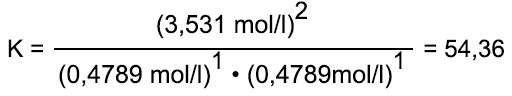The constant K = 54.36 applies to a certain temperature and increases with decreasing temperature.

Influencing the chemical equilibrium

Whether the position of equilibrium is more on the side of the products or more on the raw materials depends on several factors. In the case of very large values ​​of the equilibrium constants, the equilibrium is predominantly on the side of the products, in the case of very small values ​​on the side of the starting materials.

1.) A change in temperature leads to a shift in equilibrium. This should be illustrated by the equilibrium reaction of nitrogen dioxide to dinitrogen tetroxide:

2 NO2 (G)N2O4 (G) ΔHR. = −58.2 kJ / mol

An increase in temperature favors the endothermic partial reaction, the equilibrium shifts to the left. The partial reaction to the right would be the exothermic reaction, the partial reaction to the left would be the endothermic. Therefore, when the temperature rises, more brown nitrogen dioxide (NO2).

Lowering the temperature favors the exothermic partial reaction. When the temperature drops, the brown color decreases again and more colorless dinitrogen tetroxide is formed, the equilibrium shifts to the right.

2.) If gases are involved, a change in pressure also shifts the chemical equilibrium. If the pressure increases in a closed system of substances, the equilibrium shifts in the direction in which the substances take up less volume. Example: In ammonia synthesis, one volume fraction of nitrogen reacts with three volume proportions of hydrogen to form two volume proportions of ammonia gas:

N2 (g) + 3H2 (G)2 NH3 (G) ΔHR. = −92 kJ / mol

As a result, the equilibrium shifts to the right when the pressure increases, since less gas volume is to be expected there.

Principle of Le Chatelier

In 1884, the French chemist Henry Louis Le Châtelier (1850–1936) tried to formulate a law that generally describes how an equilibrium shifts when external conditions such as temperature and pressure change. The law is as Principle of Le Chatelier or as Principle of escape from compulsion known:

If a compulsion is exerted on a system that is in equilibrium due to a change in the external conditions, the equilibrium shifts in such a way that it evades the compulsion. A new equilibrium is established with reduced coercion.

Although the principle cannot make any quantitative statements, it does make essential statements in the manufacture of chemical products in laboratories and technology.

In addition to the change in temperature and pressure, a change in the concentration of the substances involved also causes a shift in the equilibrium. According to the quotientAn addition of A or B and a withdrawal of C or D cause a shift of the equilibrium in the direction of the products C and D. This guarantees that the same value of the equilibrium constant is maintained. If a product escapes continuously as in an open system, the reaction runs almost completely towards the product side.

Summary

If you want to achieve the highest possible yield in a synthesis, then
• one changes the temperature and the pressure so that the equilibrium system evades the constraint in the direction of the products,
• if the concentration of a starting material is increased,
• you take a product away all the time or you add a catalyst as well. However, this does not change the equilibrium constant, but accelerates the setting of the equilibrium.
•
Supplementary content

Chemical equilibrium demonstrations
Ammonia synthesis

Create your own book: Basic text Chemical equilibrium